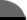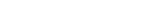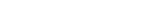Facts By Category:» Physics  » Astronomy  » Chemistry  » Biology  » Mathematics  » Geology  » Engineering  » Medicine  » Science  ScienceIQ Team:»Writers & EditorsxUmp.com
Science Supplies,

Physics & Astronomy
Online
How To Calculate The Volume Of A Right ConeCones are used every day for a variety of purposes. Perhaps the most useful application of the cone shape is as a funnel. For finding the volume, a cone is best viewed as a stack of circles, each one smaller than the one before, until the last is no more than a point on the line that passes through the center of each circle throughout the length of the cone. For a cylinder, the volume is given by a formula that multiplies the area of a circle by the height of the cylinder. A cone is just a cylinder that tapers, and the averaging formula applies to it just as well because of its regular shape. In a cylinder, the average of the area at both ends and at the center of the cylinder, multiplied by the height of the cylinder provides the volume of the cylinder. When the same calculation has been carried out for a right cone, the volume is given by the general equation V = (1/3)pr2h, where r is the radius of the base, and h is the vertical height of the cone.

As an example of how to use this equation, suppose you wish to construct a feed hopper in your chicken barn. You need it to be 2 feet in diameter and it must hold an entire bag of chicken feed, 3 cubic feet. So you need to know how long the cone-shaped hopper must be. The radius is 1 foot, and the volume is 3 cubic feet. To find the height of the cone, rearrange the volume equation and substitute these known values (use p = 3.14)

V = (1/3)pr2h, = (1/3) X 3.14 X 1 X 1 X h, therefore, h = (3 X V) / (3.14 X 1 X 1), = (3 X 3) / 3.14, = 9 / 3.14, = 2.86. So if you make the hopper 3 feet high, it will be more than big enough.

477Richard M. J. Renneboog, MS Richard M. J. Renneboog is an independent private technical consultant and writer in both chemical and computer applications. Endeavors have included preparation of scripts for instructional and promotional video, corporate website design, curriculum development for training in advanced composites technology, and development.Geometry of Design: Studies in Proportion and Compositionby Kimberly Elam
 Home | Privacy Policy | Cookie PolicyCopyright © 2002-2019 ScienceIQ.com - All Rights Reserved